# Lab 6 - Bootstrap and PermutationFeb 13 2019

library(faraway)
library(tidyverse)

The code in today’s lab comes straight from Chapter 3 in Linear Models with R with only minor edits that I think improve clarity.

# Preliminaries

## Brief review of for loops

An example of a for loop in R:

n_sims <- 10
output <- numeric(n_sims)
for (i in 1:n_sims){
output[i] <- mean(rnorm(20))
}
output
##   -0.21798434 -0.16131401  0.50563841 -0.25441329  0.14596754
##    0.18129472 -0.14947953  0.02673052  0.09009637 -0.12237762

TA to remind you about parts of a for loop:

• Output
• Sequence
• Body

There are alternatives to for loops that remove all the repetitive book-keeping parts and let you focus on what is being done rather than how it is being done, for example the map() functions in the purrr package. We won’t use them here, because when you see these statistical methods first, I think the for loop syntax more closely matches the textual description of what is happening (at least how Faraway describes it).

## The sample() function

Used in two ways (in Chapter 3 in Faraway):

1. To randomly permute a set of values:

sample(1:5)
##  3 4 2 1 5
sample(c("a", "b", "c"))
##  "a" "c" "b"

With no further arguments, sample() will randomly reorder the vector you give it.

2. To sample with replacement from a set of numbers, add replace = TRUE:

sample(1:5, replace = TRUE)
##  4 3 3 3 5
sample(c("a", "b", "c"), replace = TRUE)
##  "a" "c" "c"

In both cases we are omitting the size argument, which by default is the same as the length of the input vector.

# Bootstrap confidence intervals

Overall idea: To understand the sampling distribution of a parameter estimate, generate data that approximate what repeated experiments/samples would look like.

Procedure From Faraway (Model based bootstrap)

Repeat many times:

1. Generate $$\epsilon^*$$ by sampling with replacement from the residuals, $$e_1, \ldots, e_n$$.
2. Form $$y^* = X\hat{\beta} + \epsilon^*$$.
3. Compute $$\hat{\beta}^*$$ from $$(X, y^*)$$.

Examine distribution of parameter estimates generated by bootstrap to make inferences on true parameter value.

## Setup

Model based bootstrapping requires us to assume a specific model form, fit it:

lmod <- lm(Species ~ Area + Elevation + Nearest + Scruz + Adjacent,
data = gala)

And retain the fitted values and residuals:

resids <- residuals(lmod)
preds <- fitted(lmod)

## A single bootstrap sample

A single bootstrap dataset is constructed by adding the fitted values (i.e. $$X\hat{\beta}$$), and bootstrapped errors (a sample with replacement from the residuals):

# bootstrapped errors
(errors <- sample(resids, replace = TRUE))
##         Eden      Isabela     Espanola     Champion SanCristobal
##   -20.314202   -39.403558    49.343513    14.635734    73.048122
##       Pinzon      Isabela    SantaCruz      Enderby     Gardner1
##   -42.475375   -39.403558   182.583587   -28.785943    62.033276
##       Pinzon     Champion    Bartolome        Pinta      SantaFe
##   -42.475375    14.635734    38.273154  -111.679486   -23.376486
##        Pinta    Bartolome     Genovesa      Isabela     Espanola
##  -111.679486    38.273154    40.497176   -39.403558    49.343513
##       Pinzon       Rabida       Baltra      Coamano    SantaCruz
##   -42.475375    -5.553122   -58.725946    38.383916   182.583587
##       Darwin     Caldwell        Pinta      Tortuga      Seymour
##    19.018992   -26.330659  -111.679486   -36.935732    -5.805095
# bootstrapped response
preds + errors
##       Baltra    Bartolome     Caldwell     Champion      Coamano
##   96.4117443  -46.6767121   78.6741729   25.0000000   36.6642065
## Daphne.Major Daphne.Minor       Darwin         Eden      Enderby
##    0.6123302   -5.4838900  173.5645950   -0.4717408   92.8192184
##     Espanola   Fernandina     Gardner1     Gardner2     Genovesa
##    5.1811115  111.6253322   34.2398785  -47.0456905  -23.8736612
##      Isabela     Marchena       Onslow        Pinta       Pinzon
##  274.7240716  126.9676948   44.5344083  176.2759284  199.8188885
##   Las.Plazas       Rabida SanCristobal  SanSalvador    SantaCruz
##   -7.3995684   70.0000000  148.2259319  316.0602339  444.0000000
##      SantaFe   SantaMaria      Seymour      Tortuga         Wolf
##  104.3954776  169.2859692  -61.8743916   16.0000000   20.8954789

In this example our model involves multiple terms so it’s a little hard to visualise. But here are the original fitted values (blue open dots) along with the a bootstrapped set of response values (black filled dots) against one of the predictors, Elevation:

ggplot(gala, aes(Elevation, preds)) +
geom_point(color =  "#377EB8", shape = 21) +
geom_point(aes(y = preds + errors), color = "black")+
ylim(c(-200, 500))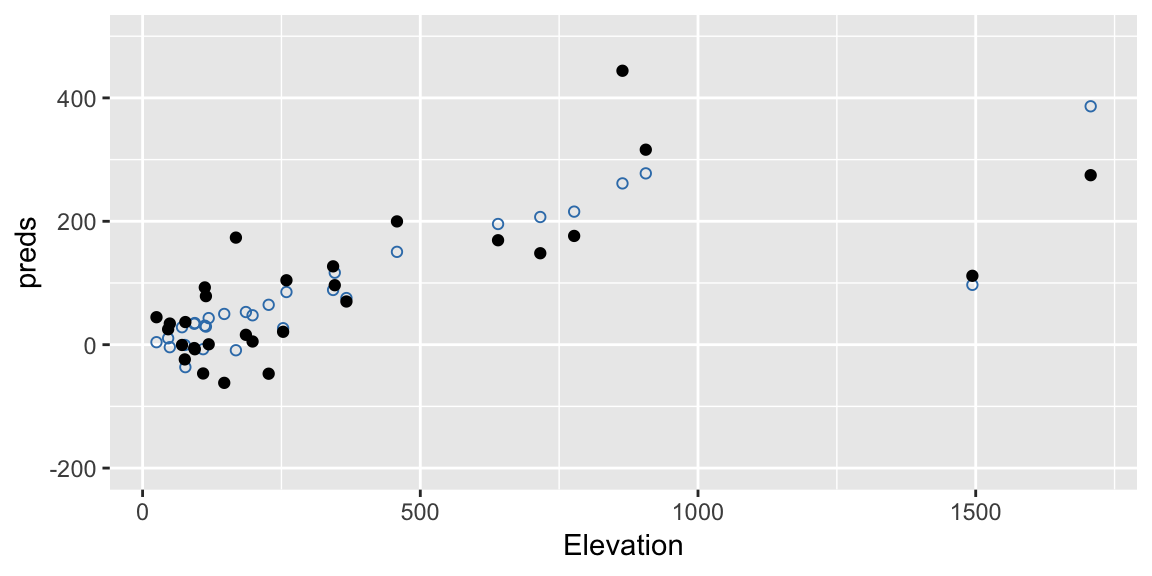Here’s another one:

errors <- sample(resids, replace = TRUE)
ggplot(gala, aes(Elevation, preds)) +
geom_point(color =  "#377EB8", shape = 21) +
geom_point(aes(y = preds + errors), color = "black") +
ylim(c(-200, 500))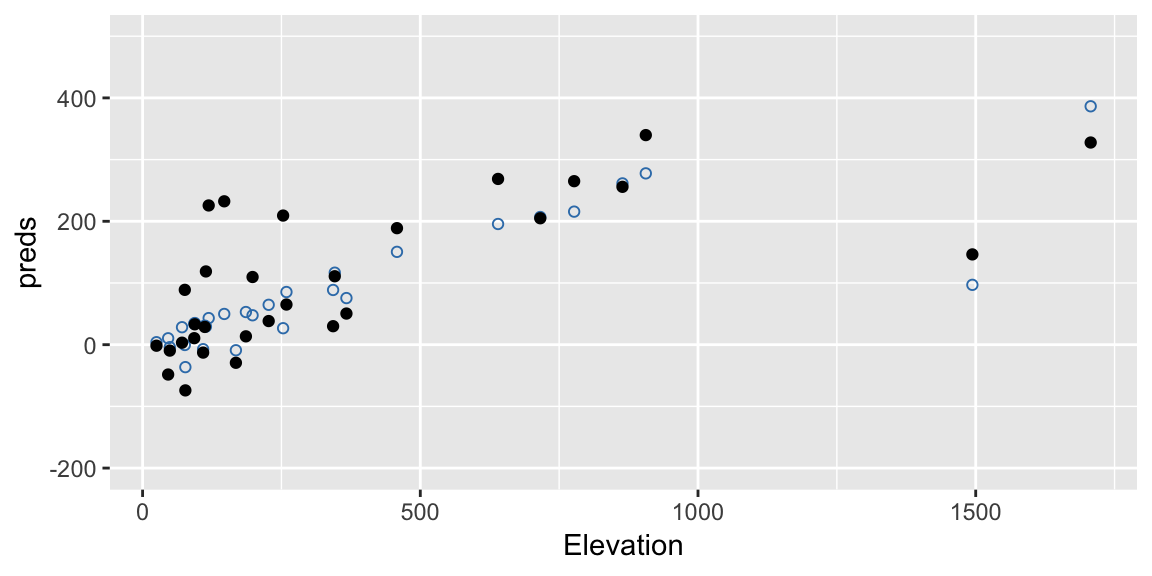Notice this process retains the structure implied by the fitted model. So, if our model has a large effect due to Elevation, we should maintain that effect in our bootstrap samples.

## Repeating many times

We can repeat this process many times each time fitting the model and retaining the estimated coefficients:

nb <- 4000
coefmat <- matrix(NA, nrow = nb, ncol = 6)
colnames(coefmat) <- names(coef(lmod))
for (i in 1:nb){
booty <- preds + sample(resids, replace = TRUE)
bmod <- update(lmod, booty ~ .)
coefmat[i, ] <- coef(bmod)
}

Then using these many estimated coefficients we can construct confidnce intervals using the percentile method:

(cis <- apply(coefmat, 2, quantile, probs = c(0.025, 0.975)))
##       (Intercept)        Area Elevation   Nearest      Scruz    Adjacent
## 2.5%    -25.04466 -0.06304558 0.2259949 -1.683388 -0.6046124 -0.10553011
## 97.5%    41.41317  0.02103163 0.4198246  2.139087  0.1760862 -0.03806448

Or create density plots on individual parameters:

coefmat <- as_tibble(coefmat)
cis <- as_tibble(cis)

ggplot(coefmat, aes(x = Area)) +
geom_density() +
geom_vline(aes(xintercept = Area), data = cis, linetype = "dashed",
color = "grey20") +
labs(title =
expression(paste("Bootstrap estimate of the sampling distribution for ", hat(beta)[Area])))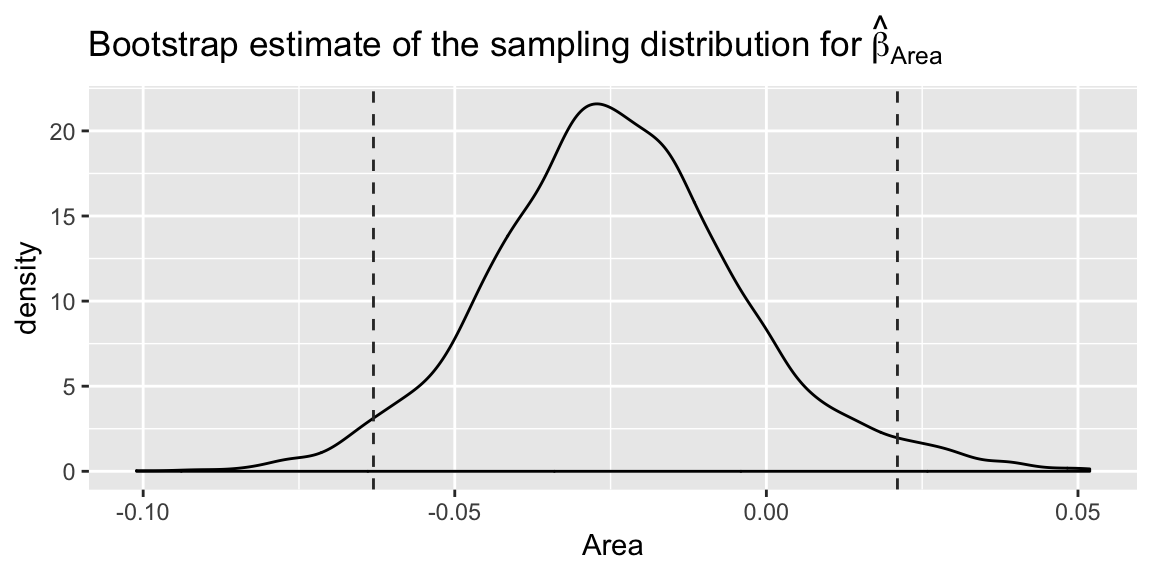# Permutation tests

Overall idea: To understand the null distribution of a test statistic, approximate what repeated experiments/samples would look like, if the null hypothesis was true.

Procedure

Repeat many times:

1. Generate $$(X^*, y^*)$$ by permutation, in a way suggested by the null hypothesis.
2. Compute test statistic $$T(X^*, y^*)$$.

Compare observed test statistic to the distribution of test statistics generated by permutation, to conduct a hypthothesis test.

## An overall F-test example

Faraways chooses an example with a not too small overall F-test p-value (I’ve given this model object a different name to Faraway to emphasize this is not the same model as lmod above):

lmod_small <- lm(Species ~ Nearest + Scruz, data = gala)
lms <- summary(lmod_small)

The overall F-test statistic is available:

lms$fstatistic ## value numdf dendf ## 0.6019558 2.0000000 27.0000000 which we can use to find the corresponding p-value: 1 - pf(lms$fstatistic, lms$fstatistic, lms$fstatistic)
##     value
## 0.5549255

This p-value is based on our assumption of Normal errors. We’ll save the statistic for later:

obs_fstat <- lms$fstat The permutation approach attempts to find the null distribution of this test statistic without relying on any distributional assumptions. The null hypthothesis in this overall F-test, is that none of the explanatory variables impact our response. If this was the case, the response value we see for a particular island shouldn’t depend on its attributes. We should have been just as likely to see 58 species on Bartolome and 31 species on Baltra instead of the other way around. In fact, any permutation of the response values assigned to islands should have been equally likely to occur. (Notice this feels a little awkward with observational data. It is generally much easier to justify a permutation approach in a randomized experiment, where the treatment variables were actually assigned to units at random.) ## A single permutation To generate a null distribution of the test statistic we consider permutations of the response variable. permuted_species <- sample(gala$Species)

Again its hard to visualise becasue we have a few variables involved, but take a look at this permuted response (black filled points) against Elevation:

ggplot(gala, aes(Elevation, Species)) +
geom_point(color = "#377EB8", shape = 21) +
geom_point(aes(y = permuted_species), color = "black") +
ylim(c(-200, 500))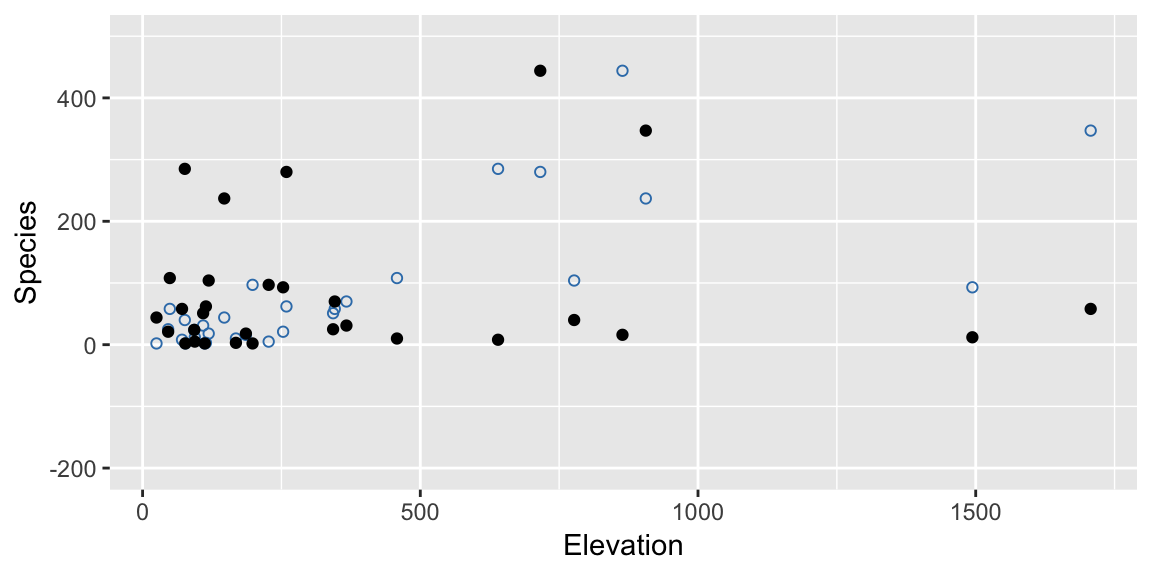Notice that a key difference between this and the bootstrap approach is that we break relationships here. In the original data there was a strong relationship between Elevation and Species, but under the null hypothesis there isn’t, and our permutated data reflects this.

To get a test-statistic from this data, we fit the model of interest and extract the F-statistic:

lm_permutation <- lm(permuted_species ~ Nearest + Scruz, data = gala)
summary(lm_permutation)$fstat ## value ## 7.434452 This is an example of one test statistic generated under the null hypothesis, to conduct a test we need a whole lot of them. ## Scaling up nperms <- 4000 fstats <- numeric(nperms) for (i in 1:nperms){ lmods <- lm(sample(Species) ~ Nearest + Scruz, data = gala) fstats[i] <- summary(lmods)$fstat
}

These statistics then form our null distribution:

ggplot(mapping = aes(x = fstats)) +
geom_density() +
geom_vline(aes(xintercept = obs_fstat), linetype = "dashed",
color = "grey20") +
labs(title = "Permutation based null distribution for the overall F-statistic",
xlab = "F-statistic")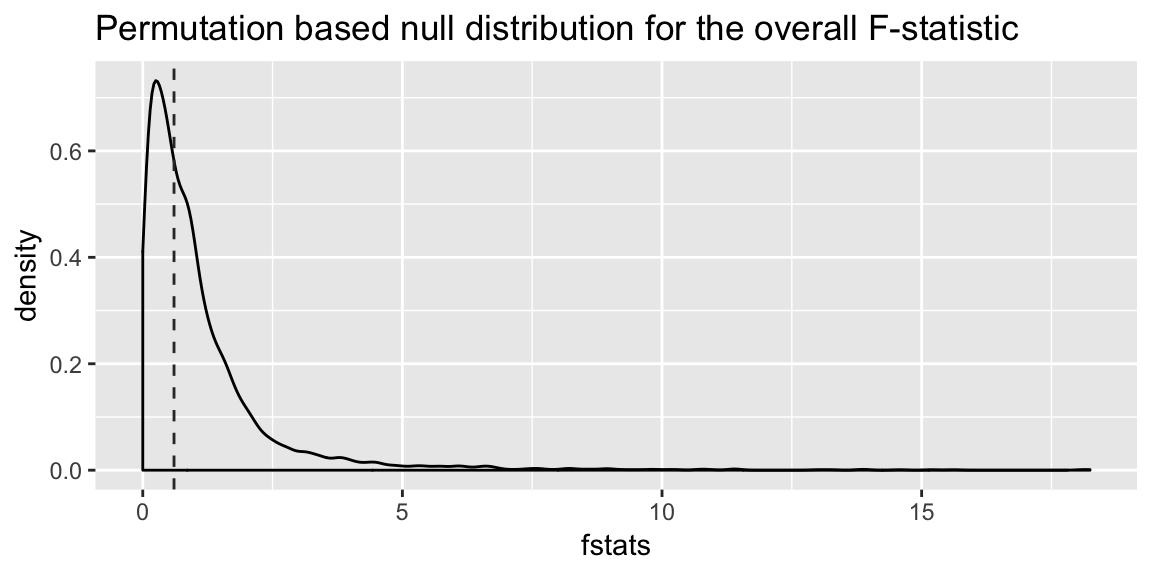And to get a p-value, we find the proportion of test statistics generated under the null hypothesis that are more extreme than that observed:

mean(fstats > obs_fstat)
##  0.5565

In this case, pretty close to the p-value derived using the Normal assumptions.

## An individual parameter example

You can also use a permuation approach to test individual parameter estimates in a regression. Now, since the null hypothesis is only about one parameter, after accounting for the effects of the other variables, we only permute values of the explanatory varibale of interest.

For example, in lmod_small we might ask only about the parameter on Scruz, $$\beta_{\text{Scruz}}$$. For the null hypothesis $$\beta_{\text{Scruz}} = 0$$, the Normal based inference gives us a p-value of 0.28:

summary(lmod_small)$coef ## Estimate Std. Error t value Pr(>|t|) ## (Intercept) 98.4765494 28.3561013 3.472852 0.001751461 ## Nearest 1.1792236 1.9184475 0.614676 0.543914527 ## Scruz -0.4406401 0.4025312 -1.094673 0.283329519 We’ll still the t-statistic as our test statistic, so we’ll keep the value from the observed data: obs_tstat <- summary(lmod_small)$coef[3, 3]

But now we’ll use permutation to find a null distribution rather than relying on theory and comparing it to a Student’s t-distribution.

tstats <- numeric(nperms)
for (i in 1:nperms){
lmods <- lm(Species ~ Nearest + sample(Scruz), data = gala)
tstats[i] <- summary(lmods)$coef[3, 3] } Here’s the resulting null distribution, with the t-distribution that the Normal assumption would lead to overlaid: ggplot(mapping = aes(x = tstats)) + geom_density() + geom_vline(aes(xintercept = obs_tstat), linetype = "dashed", color = "grey20") + stat_function(fun = dt, args = list(df = lmod_small$df.residual),
color= "grey50")+
labs(title = expression(
paste("Permutation based null distribution for the t-statistic, ", beta[Scruz]==0)),
xlab = "t-statistic")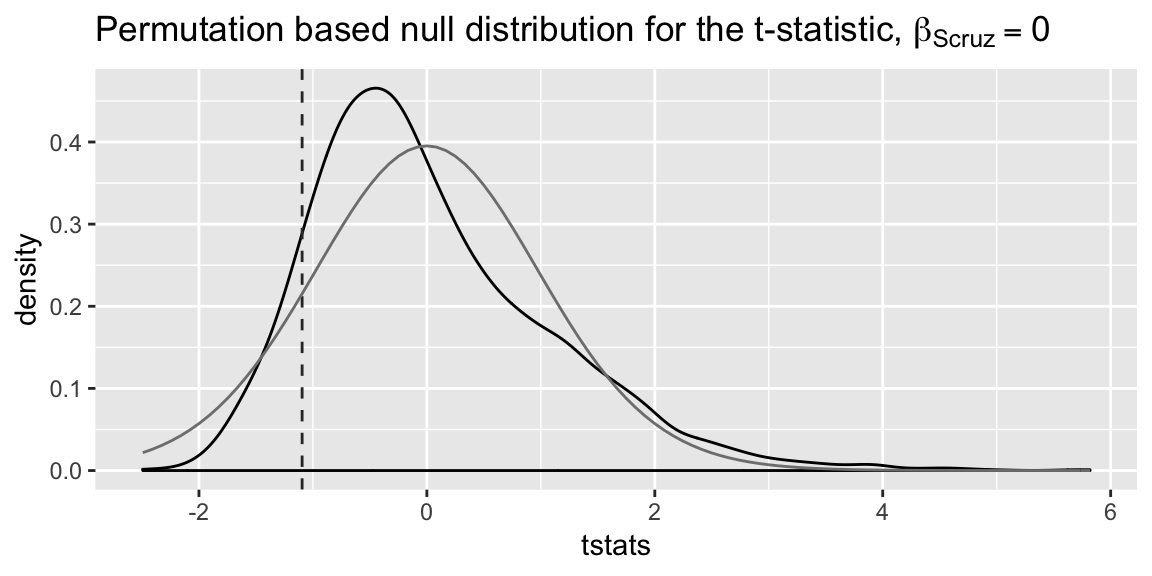Notice the null distribution based on the permutation test doesn’t seem that close to the t-distribution. However, the p-value isn’t particularly different in this case:

mean(abs(tstats) > abs(obs_tstat))
##  0.26375